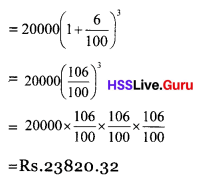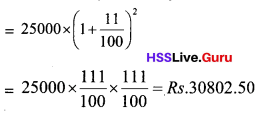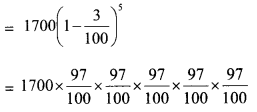# Kerala Syllabus 8th Standard Maths Solutions Chapter 5 Money Maths

## Kerala State Syllabus 8th Standard Maths Solutions Chapter 5 Money Maths

### Money Maths Text Book Questions and Answers

Textbook Page No. 90

Money Maths Class 8 Question 1.
Sandeep deposited Rs 25000 in a bank which pays 8% interest compounded annually. How much would he get back after two years?
Solution:
Interest in the first year
= 25000 × $$\frac{8}{100}$$
=Rs 2000
Principal in the second year
= 25000 + 2000
= 27000
Interest in the second year
= 27000 × $$\frac{8}{100}$$
= 2160
Total amount gets back at the end of 2 year
= 27000 + 2160
= 29160

Question 2.
Thomas took out loan of 15000 rupees from a bank which charges 12% interest, compounded annually. After 2 years, he paid back 10000 rupees. To settle the loan, howmuch should he pay at end of three years?
Solution:
Amount borrowed = 15000
Interest for the first year
= 15000 × $$\frac{12}{100}$$ = 1800
Loan for the second year
= 15000 + 1800 = Rs.16800
Interest for the second year
= 16800 × $$\frac{12}{100}$$ = 2016
Loan for the end of second year
= 16800 + 2016 = 18816
Amount payback at the end of second year = 10000
Loan for the third year
= 18816 – 10000 = 8816
Interest for the 3rd year
= 8816 × $$\frac{12}{100}$$ = 1057.92
Amount to be repair at the end of third year
= 8816 + 1057.92 = Rs.9873.92

Question 3.
Rs 200 was got as interest from a bank for 2 years at the rate 5%. Compute the compound interest for the same principal for 2 years at the same rate of interest.
Solution:
Interest for 2 years = Rs 200
Interest for one year = Rs 100
Excess amount in the 2nd year as the compound interest
= 100 × $$\frac{5}{100}$$ = 5
Compound interest for two years = Rs. 205

Textbook Page No. 92

Question 1.
Anas deposited Rs 20000 in a bank where compound interest in computed 6% annually. How much amount he will get at the end of 3rd year?
Solution:
Amount Anas gets at the end of third yearQuestion 2.
Diya Deposited Rs. 8000 in a bank where compound interest is computed annually at 10%. Rs. 5000 was withdrawn by her at the end of 2 years. How much amount will he there in the account of Diya after 1 more year?
Solution:
Amount deposited = 8000
Rate of interest = 10%
Amount in the account of Diya at the end of 2 years
= 8000 × (1 + $$\frac{10}{100}$$)2
= 8000 × $$\frac{110}{100}$$ × $$\frac{110}{100}$$ = Rs.9680
Amount withdrawn =Rs. 5000
Principal for the 3rd year
= 9680 – 5000 = Rs.4680
Amount she get at the end of 3 rd year
= 4680 (1 + $$\frac{10}{100}$$)
= 4680 × $$\frac{110}{100}$$
= Rs. 5148Question 3.
Varun borrowed rupees 25000 from a bank where compound interest in computed at 11% annually. He repaid Rs 10000 at the end of 2 years. How much amount he has to repay after one more year?
Solution:
Amount borrowed = 25000
Rate of interest = 11%
Total liability after 2 yearAmount he repaired = Rs.10000
Principal for the third year
= 30802.50 – 10000
= Rs.20802.50
Amount he has to repay at the end of third year
= 20802.50 × (1 + $$\frac{11}{100}$$)
= 20802.50 × $$\frac{111}{100}$$
= Rs. 23090.78

Textbook Page No. 93

Question 1.
Arun deposited Rs 5000 in a bank where compound of interest is computed half yearly. Mohan deposited RS 5000 in a bank where compound interest is computed quarterly she rate of interest at both the banks is 6%. Money was withdrawn by both of them after an year. How much amount Mohan got more than Arun.
Solution:
Amount deposited by Arun = 5000.
Rate of interest = 6%
Amount Arun gets after an year
= 5000 × (1 + $$\frac{8}{100}$$)2
= 5000 × $$\frac{103}{100}$$ × $$\frac{103}{100}$$
= 5304.50
Amount deposited by Mohan
= Rs 5000
Rate of interest = 6%
Amount Mohan gets after 1 year= Rs 5306.82
Amount Mohan gets more than
Arun = 5306.82 – 5304.50
= Rs 2.32

Question 2.
Rs. 16000 was borrowed by a man from a bank where compound interest is computed quarterly. Annual interest rate is 10%. How much he has to pay after 9 months to clear his liabilities?
Solution:
Amount borrowed = Rs 16000
Interest rate = 10 %
9 Months = 3 quarterly years
Amount to be repaired after 9 months
= 16000 × (1 + $$\frac{2.5}{100}$$)3
= 16000 × ($$\frac{102.5}{100}$$)3
= 16000 × $$\frac{102.5}{100}$$ × $$\frac{102.5}{100}$$ × $$\frac{102.5}{100}$$
= 17230.25

Question 3.
Manu deposited Rs 15000 in a financial institution. Interest is calculated in every 3 months and added to the amount. Rate of interest in 8 %. How much he gets after 1 year.
Solution:
Amount deposited = Rs 15000
Rate of interest = 8%
Amount he gets after 1 yearQuestion 4.
John deposited Rs 2500 on 1st January in a co-operative bank. Bank computes compound interest half yearly. Annual interest rate is 6%. Again he deposited Rs 2500 on 1st July? How much amount he will have in his account at the end of the year?
Solution:
Amount John deposits on 1st January = Rs.2500
Principal after the half year up to July
1 st = 2500 (1 + $$\frac{3}{100}$$)
= 2500 × $$\frac{103}{100}$$
= Rs.2575
Amount deposited in July 1st
= Rs 2500
Principal after July 1st= 2575 + 2500 = 5075
Amount he gets at the end of the year 3
= 5075 × (1 + $$\frac{3}{100}$$)
= 5075 × $$\frac{103}{100}$$
= Rs 5227.25Money Maths Class 8 Question 5.
Ramlath deposited Rs 30,000 in a financial institution where compound interest is computed in every four months. The ann¬ual interest rate in 9%. How much amount Ramlath gets after 1 year?
Solution:
Amount deposited =Rs 30,000
Rate of interest = 9 %
Amount gets after 1 year
= 30000 (1 × $$\frac{3}{100}$$)3
= 30000 × $$\frac{103}{100}$$ × $$\frac{103}{100}$$ × $$\frac{103}{100}$$
= Rs.32781.81

Textbook Page No. 95

Compounded Half Yearly Question 1.
The e-waste increases by 15 % every year, according to the study report. There was around 9 crore tonnes of e-waste in 2014. Then how many tonnes of e-waste will be there in 2020.
Solution:
E-waste in 2014 – 9 crore tonnes
Rate of increase = 15 %
The E-waste in 2020 = 9 × (1 + $$\frac{15}{100}$$)6
= 9 × ($$\frac{115}{100}$$)6
= 20. 82 crore tonnes

Question 2.
A T.V manufacturer reduces the price of a particular model by 5% every year. The current price of this model is Rs. 8000. What would be the price after 3 years?
Solution:
The present price of the T.V = Rs. 8000
rate of reduction = 5% ,
Price after 2 years = 8000 (1 – $$\frac{5}{100}$$)2
= 8000 × ($$\frac{95}{100}$$)2
= 8000 × $$\frac{95}{100}$$ × $$\frac{95}{100}$$
= Rs.7220

Question 3.
Tiger is our national animal. Ac-cording to a statistics the number of tigers is reduced annually by 3%. There are 1700 tigers in 2011 according to the senses or tiger protection authority. Then how many tigers will be there in 2016?
Solution:
The number of tigers in 2011 = 1700
Reduction rate = 3%
No. of tigers in 2016 after 5 years of the senses= 1459.84
= 1460

Question 1.
Nirmala deposited Rs. 20,000 in a bank where compound interest of rate 7% is computed annually Rs. 5000 was with drawn after one year. How much amount she will get after 2 years.
Solution:
Amount deposited in the bank = Rs. 2000
Rate of interest = 7%
principal after one year
= 20000 + [20000 × $$\frac{7}{100}$$] = 20000 + 1400 = 21400
Amount withdrawn after one year = Rs. 5000 .
Principal for the 2-nd year
= 21400 – 5000 = Rs. 16400
Amount she gets after 2 years
= 16400 + [16400 × $$\frac{7}{100}$$] = 16400 + 1148
= Rs. 17548

Question 2.
Aswathy deposited Rs. 10,000 in a bank where compound interest is calculated at the rate of 10%. Rs.5000 was deposited in the beginning of the 2-nd year and Rs.5000 in the beginning of the 3-rd year. How much amount she gets after 3 years?
Solution:
Amount deposited in the first year = Rs. 10,000
rate of interest = 10%
Amount at the end of first year
= 10000 + [10000 × $$\frac{10}{100}$$] = 10000 + 1000 = Rs. 11000
Principal’for the 2-nd year
= 11000 + 5000 = Rs. 16000
Amount at the end of the 2-nd year
= 16000 + [16000 × $$\frac{10}{100}$$] = 16000 + 1600 = Rs. 17600
Principal for the 3-rd year
= 17600 + 5000 = Rs.22600
Amount at the end of 3-rd year
= 22600 + [22600 × $$\frac{10}{100}$$] = 22600 + 2260 = Rs. 24860
Amount Aswathy will get at the end of 3-rd year = Rs. 24860

Question 3.
Raj an borrowed Rs. 15000 from a co-operative bank for business purpose. The bank computes 9% interest. How much money she has to repay after 5 months.
Solution:
Amount borrowed = Rs. 15000
Rate of interest = 9%
Time duration = 5 month months
Interest .
= 15000 × $$\frac{9}{100}$$ × $$\frac{5}{12}$$ = 562.50
Amount he has to repay
= 15000 + 562.50
= Rs 15562.50

Question 4.
Rasiya deposited Rs. 20,000 in a bank where compound interested is computed half yearly. If the rate of interest is 11%, How much amount she will get after an year?
Solution:
Capital for first year = Rs. 20,000
Rate of interest = 11 %
Interest of first half year
= 20000 × $$\frac{11}{100}$$ × $$\frac{1}{2}$$ = Rs. 1100
Principal of 2-nd half year
= 20000 + 1100
= 21100
Interest of the 2-nd half year
= 21100 × $$\frac{11}{100}$$ × $$\frac{1}{2}$$ = Rs. 1160.5
Amount she gets after one year
= 21000 + 1160.5
= Rs. 22160.5

Question 5.
The price of a car is rupees 5 lakh and it depreciates by 6% every year. What would be the price after 2 year ?
Solution:
Here the price every year is 6% less than the previous years price.
First year’s price = Rs 500000
First year’s depreciation
= 500000 × $$\frac{6}{100}$$ = Rs. 300000
Second year’s price = Rs 4,70000
Second year’s depreciation
= 470000 × $$\frac{6}{100}$$ = 28200
The price of the car after 2 years
= Rs 4,70,000 – Rs 28200
= Rs 441800

Question 6.
The simple interest of an amount is Rs 50 for two years and the compound interest is Rs 55. The rate of interest is same in both the cases. Find the rate? Find the amount?
Solution
The simple interest of the amount for two years = Rs 50
The simple interest of the amount for 1 year = Rs 25
The compound interest of the amount for 2 years = Rs 55
The interest in the 2 nd year
= 55 – 25 = Rs 30
Interest for Rs 25 = Rs 5Question 7.
A company which manufactures computers increases its production by 10% every year. In 2009 the company produced 80,000 computers. How many computers would it produced in 2011?
Solution:
Here the number of computers produced every year is 10% more than the number produced the year before. So starting from 80,000. We have to find the number of computers produced every year after that for two years.
Number of computers produced in 2009 = 80,000
Number of computers produced in 2010
= 80000 + 80000 × $$\frac{10}{100}$$
= 80000 + 8000 = 88000
Number of computers produced in 2011
= 88000 + 88000 × $$\frac{10}{100}$$
= 88000 + 8800 = 96800

Question 8.
Ramu borrowed Rs 50,000 from a bank at the interest rate of 10% for agricultural purpose. The interest rate will be reduced by 5% if he repays the amount properly within 2 years. If he fails to repay in time there will be fine as 1%. Ramu could not repay the amount in time. How much amount Ramu repair?
Solution:
Amount borrowed =Rs 50,000
Rate of interest = 10%
Interest rate including fine = 10 + 1=11%
Amount to be repaid
= 50000 + [50000 × $$\frac{11}{100}$$ × 2]
= 50000 + 11000 = 61000
= Rs 61000Question 9.
Raju has Rs 800 with him. He spent 25 % of it. How much amount left with him?
Solution:
Total amount = Rs 800
Amount spent = 800 × $$\frac{25}{100}$$ = 200
Amount left with Raju = 800 – 200
= Rs 600

Question 10.
Balu and Ramu decided to borrow Rs 15000 each for a joint business. Balu borrowed Rs 15000 from a financier Who imputes Rs 5 per month for Rs 100 as interest. Ramu borrowed Rs 15000 from a bank where compound interest of 12 % is computed. How much money both of them have to repay after 2 years?
Solution:
Interest Balu has to pay after 2 years
= 15000 × $$\frac{60}{100}$$ × 2 = Rs. 18000
(Interest for Rs 100 per month in Rs 5. Interest for Rs 100 in an year = 5 × 12
= Rs. 60.
The rate of interest = 60%)
Amount Balu has to repay after 2 years
= 15000 + 18000
= Rs 33000
In the case of Ramu interest is computed as compound interest.
Principal for first year = Rs 15000
Interest for 1 st year
= 15000 × $$\frac{12}{100}$$ × 1 = Rs 1800
Principal for 2 nd year
= 15000 + 1800
= Rs 16800
Interest for the second year = 16800 × $$\frac{12}{100}$$ × 1 = Rs. 2018
Amount Ramu has to repay
= 16800 + 2018
= Rs 18816

Question 11.
The population of Kerala increases by 3% every year. The current population is 5 crore. What would he the population after 2 years.
Solution:
Current population = 50000000
Percentages increase in every year = 3%
The population in Kerala after 3 year
= 50000000 [1 + $$\frac{3}{10}$$]2
= 50000000 × $$\frac{(103)^{2}}{10000}$$
= 530,45000

Question 12.
A financial company claims that it charges only 20% interest on loans. But if a person takes out a loan of Rs 100 he would get only Rs 80, after subtracting the annual interest of rupees 20 at the outset. And he has to pay back Its 100 after 1 year. How much is their real interest?
Solution:
A borrower gets only Rs 80 when he borrows Rs 100
He has to repay Rs 100.
$$\frac{20}{80}$$ × 100 = 25%